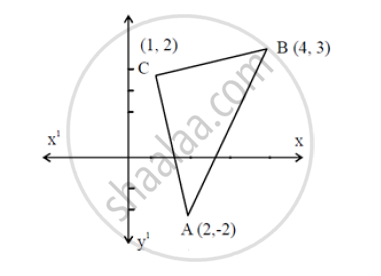# Using the Method of Integration, Find the Area of the Triangular Region Whose Vertices Are (2, -2), (4, 3) and (1, 2). - Mathematics and Statistics

Using the method of integration, find the area of the triangular region whose vertices are (2, -2), (4, 3) and (1, 2).

#### SolutionEquation of line AB : -

y+2=(2+3)/2(x-2)

⇒ 2y = 5x - 14

Equation of line BC : -

y-3=1/2(x-4)

⇒ 3y = x + 5

Equation of line CA : -

(y - 2) = - 4 (x - 1)

4x + y = 6

∴ ar (ΔABC)

=int_(-2)^3(2y+14)/5dy-int_2^3 3y-5dy

=75/5-5/2-24/4

=(300-120-50)/20=130/20

=13/2

Concept: Area of the Region Bounded by a Curve and a Line
Is there an error in this question or solution?